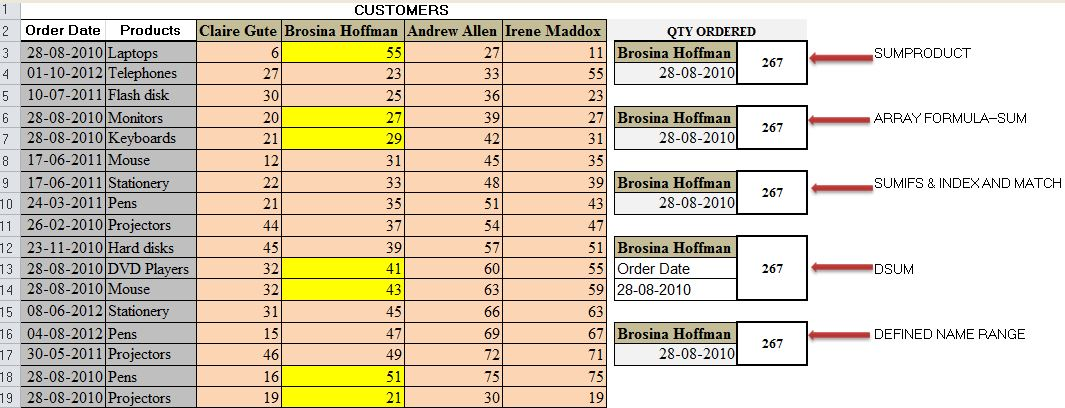#### Email Us

Follows

The ability to lookup values is a MUST have skills for all Excel users. If you cannot lookup you cannot excel in data analysis.

In this article, I will show you   ways you can do a 2-way lookup in Excel.

## 1. USING SUMPRODUCT

Using below sales data lookup and sum the total quantity for ALL items ordered by Brosina Hoffman on 28-08-2010?`=SUMPRODUCT((Order_dates=H4)*(Customers=H3)*Ordered_Quantity)=267`

NB: Below are the references to the named ranges

Order_dates = A3:A19

Customers = C2:F2

Ordered_Quantity =C3:F19

### How this works;

(Order_dates=H4) creates a Boolean array showing TRUE for the Date criteria.

```{TRUE;FALSE;FALSE;TRUE;TRUE;FALSE;FALSE;
FALSE;FALSE;FALSE;TRUE;TRUE;FALSE;FALSE;FALSE;TRUE;TRUE}```

(Customers=H3) creates a Boolean array showing TRUE for the Customer criteria.

`{FALSE,TRUE,FALSE,FALSE}`

SUMPRODUCT converts the two Boolean arrays into its numeric equivalent of 1 & o and then using the techniques of the conjunction truth table, adds up the data in the intersections 55+27+29+41+43+51+21 = 267

How to get sum total orders for more than one customer?

What is the total quantity of ALL items ordered by Brosina Hoffman & Irene Maddox on 28-08-2010?

`=SUMPRODUCT(((Customers=H4)+(Customers=H3))*(Order_dates=H5)*Ordered_Quantity)`

The trick is including the 2 customers in the function

(Customers=H4)+(Customers=H3)–this ensures the two columns are dynamically selected.

`{0,1,0,1}`

## 2. USING ARRAY FUNCTION–SUM

`{=SUM((Order_dates=H7)*(Customers=H6)*Ordered_Quantity)} =267`

The array functions follow the same truth table logic. i.e. Forms a matrix where the criteria are met and multiplies it with ordered quantity matrix and sums the totals

## 3. SUMIFS, INDEX & MATCH

If you do not understand the logic in the conjunction truth table  and you do not like array formulas then you can use SUMIFS, INDEX & MATCH

`=SUMIFS(INDEX(Ordered_Quantity,,MATCH(H9,Customers,0)),Order_dates,H10)`

### How it works;

INDEX(Ordered_Quantity,, MATCH(H9, Customers,0)) Selects the Column to sum dynamically. This provides SUMIF with a sum range

`{55;23;25;27;29;31;33;35;37;39;41;43;45;47;49;51;21}`

►SUMIFS takes in this Sum Range, filters it further using the Date criteria and then sums the array

## 4. DSUM

`=DSUM(Data_Base,H12,H13:H14)=267`

►Data_Base refers to the whole table from A2: F19–Includes the headings

### How this works:

DSUM function sums the numbers in a column given the database it contains, column Position/Column Heading and a Criteria

```DSUM( Data_base, Column_Heading, criteria )

```

## 5. DEFINED NAMED RANGES`=SUM(selected_orders)`

The trick here is to first create a named range that will select the orders and then Sum the named range.

`selected_orders= (Order_dates=\$H\$17)*(Customers=\$H\$16)*Ordered_Quantity`

### How to Create the Selected_Order Name Range

Go to Formulas►Define Name►Type the name you want►In the refers to section Paste your range (

(Order_dates=\$H\$17)*(Customers=\$H\$16)*Ordered_Quantity)

## RELATED ARTICLES

LOOKUP AND SUM IN EXCEL

SUMPRODUCT With WILD CARDS